# Index

# Types of ML systems

There are three main categories of ML systems:

• Modalities of supervision (supervised, unsupervised, reinforcement learning)

• Learning modes (online learning, batch learning)

• Type of inference (instance-based, model-based)

These criteria are not mutually exclusive and can be combined according to the needs of the scenario: a self-driving machine could take advantage of an ML system of supervised learning, which learns through batch learning and which "thinks" thanks to a statistical model that allows it to make short-term predictions ("the machine in front is braking, I will soon have to activate the brakes").

# Supervised Learning

The most common learning mode is supervised learning. It consists of training the system through data containing the desired solution: these solutions are called "labels".

An example of a "labeled" data could be the image of a car (the actual data) with the assigned car model (the label). Once an ML system has been trained on the data and their labels, the system can input a new image and output its (supposed) label! The metrics to measure the system's "skill" in assigning the right label are various and will be examined in more detail in different guides.

The two most common tasks that can be tackled with supervised learning are classification and regression. A classification problem consists of assigning a discrete label to new input. It can be a numerical value or a category (e.g. given a picture to say if it represents a dog or a cat), but in any case, it is contained in a well-defined set of options.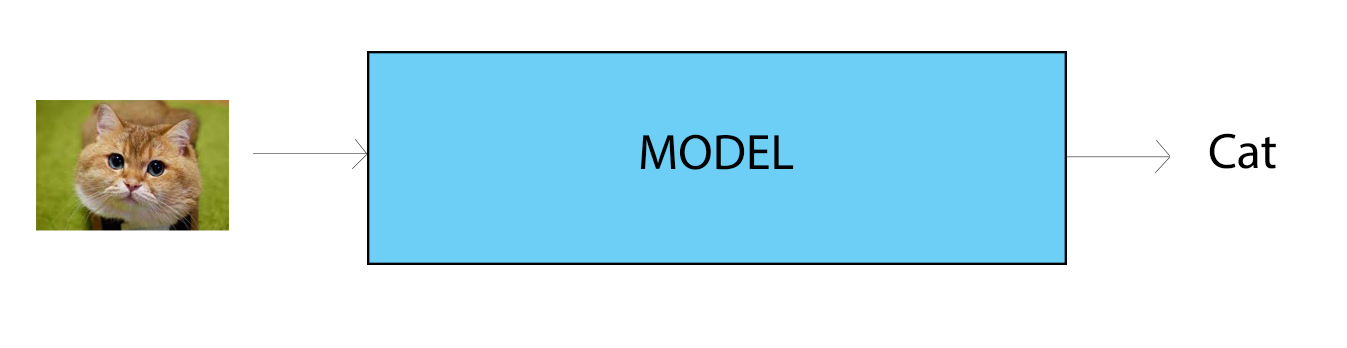A problem of regression instead consists of assigning a continuous value to the new data, for example, the price of a house given a set of values (the number of rooms for example) so the output will be included in a continuous interval and not a finite set.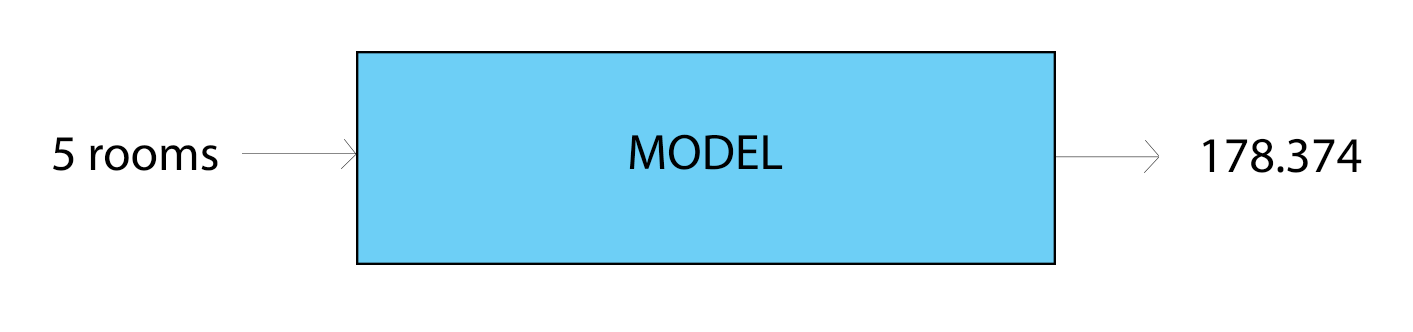Supervised learning implies a significant labeled dataset should be provided to allow the model to learn from it effectually.

The classic example is a model that is trained on a dataset of thousands of images of dogs and cats and learns to classify the new photos that are offered in one of these two categories. Classification models can learn to distinguish any number of classes, as long as they have a representative dataset!

Usually, the classification models foresee a continuous value as the probability of an input example belonging to each output class. Probabilities can be interpreted as the model's confidence that a specific example belongs to each class. A predicted probability can be converted into a class value by selecting the label of the class with the highest probability.

# Unsupervised Learning

But what if we don't have labeled data or our goal is to find something we don't know about it?

This is where unsupervised learning comes into play because the input is composed of "raw" data, without the use of labels.

For example, let's imagine that we have a supermarket sales dataset containing all the purchases made by customers, cart by cart. You can extract business advice by grouping the items often purchased together and choose to put them on nearby shelves or to discount one if you buy the others.

Unsupervised learning is also used as a complement to supervised learning to explore data (even if already labeled) and find other types of grouping that had not been noticed (and thus increase the amount of knowledge we have on each instance of the dataset).

Another use of this type of learning is for dimensionality reduction, having the purpose of reducing the number of features to consider maintaining the "value" of the data, something handy when the dataset is vast and sparse.

# Reinforcement learning

Reinforcement learning is something totally different. The learning system in this context is called agent and learns to solve the problem by observing the world around it (through some kind of sensor), performing actions, and evaluating them (good action / bad action) based on some kind of reward (reward).

The agent is designed so that it tries to improve itself, adjusting its parameters of action in action and aiming to obtain a greater and greater reward.

Reinforcement learning is used massively in robotics, where for example a robot learns to move in the surrounding environment by learning incrementally from its mistakes (the reward decreases by slamming against a wall, instead it increases while the robot moves without collisions, encouraging it to avoid objects).

# Learning modes:

Another important feature of ML systems is that they can learn either in a one-off (batch learning) mode or in a continuous incremental (online learning) mode.

In the case of batch learning (also called offline learning), the system is trained using all available data: it is usually a long and computationally expensive process, so it is performed only occasionally. When you want to re-train the model you have to do it again on all the data, so it's best to do it only if I have a considerable amount of new data, which can actually improve the performance of the new model (which will be trained on new and old ones).

Fortunately, this method of training can be easily automated so you can decide to train the model, for example, every night or every week.

However, if you need a faster system to react to changes (for example for the detection of commercial fraud or symptoms of cyber attack) the best solution is online learning. In this case (also called live learning) the system is trained sequentially by taking small batches of data, called mini-batches, as input. Learning from new data is cheap (in computational terms) and fast as the system learns from on-the-fly data as it is collected.

Online learning is optimal in cases where you need a reactive system response or you have little computational power. Note that the term "online" does not mean that the system must be connected to a geographical network, but simply be fed with a continuous flow of data.

# Type of inference:

One last way to categorize the ML systems is by how to be generalized. Model-based systems aim to create a representation of knowledge (a model), which is then used to generate outputs. Instance-based systems do not generalize from an unseen input, but compare it with all previous data (saved in memory) and find its "ideal arrangement" among them, i.e. they try to place the data at the correct point. An example can be very useful to understand this difference.

Scenario:

given a series of coordinates (datum: X, Y) that constitutes our "knowledge" try to, given the X of a new point, understand which Y is the most appropriate. A strategy could be to compare the value of X of the point with that of the known points, take the nearest known point in terms of distance, and assign its Y to the new point.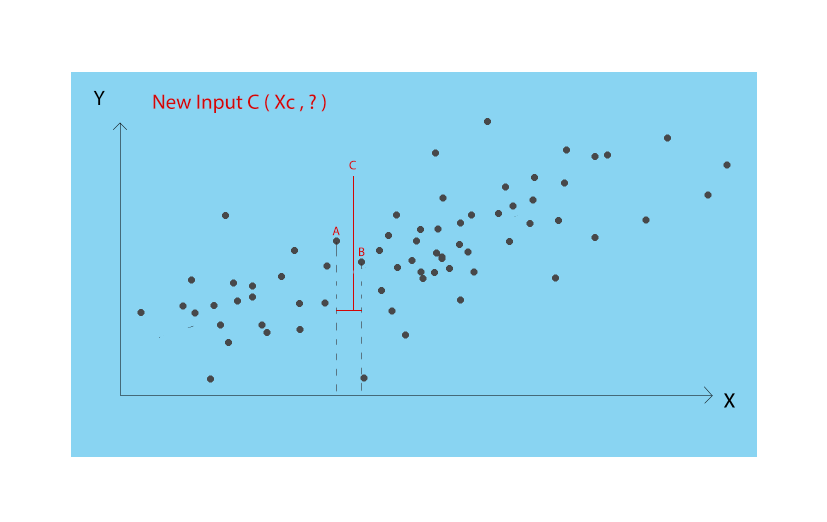However, this approach is very simplistic and is based on the strong assumption that the position of a new point is determined univocally by its closest neighbor.

The system learns its knowledge "by heart" and applies it blindly to new cases, applying some measure of similarity (in this case, the distance relative to the dimension X) concerning those stored.

The other approach that can be used to generalize new cases starting from the old ones, could be to create a "representation" or "idea" of how the data I have been made (the grey dots in figure) and use this "idea" (the model) to produce the Y of the new points. A model is, therefore, a set of parameters that, when properly adjusted, can provide a reasonable estimate of Y, given the X of a new point.

In our case, we imagine that our model is the straight line that best approximates the series of gray points. Our parameters are the slope and the intercept of the line, and the training process of the model consists in understanding what the numerical values of these two parameters are.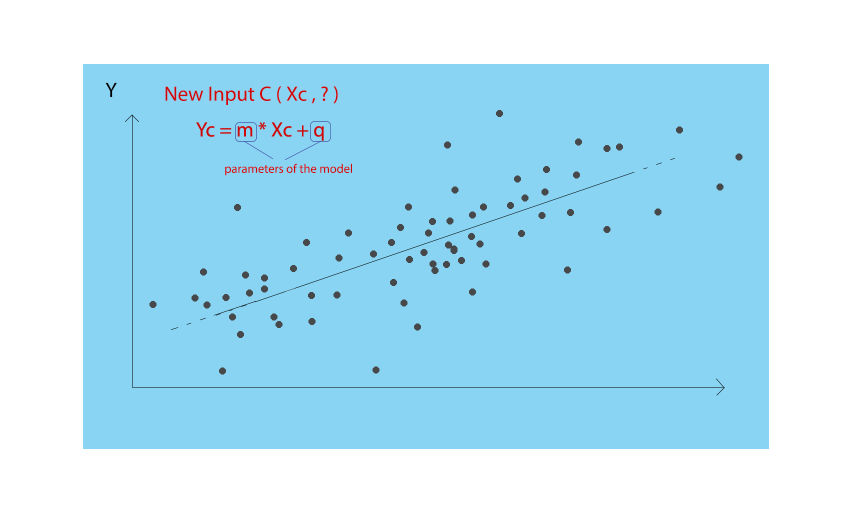A Machine Learning model, here in its simplest version (two parameters), sometimes consists of tens of thousands or even millions of parameters. To train them, and therefore find the "good values" of the parameters, a lot of computing power is needed and the optimization of the process of training the models is a heartfelt and urgent topic of research.

# Main challenges of Machine Learning

# Insufficient amount of data

The key assumption of Machine Learning is that you have the data you need to train models and use them later to solve problems. It may happen that in the real world the data you have is not enough to train a model to accurately recognize patterns that might be interesting for the problem.

Even for simple problems, thousands of examples are needed, and for complex problems such as image recognition or voice recognition, millions of examples may be needed.

Various organizations are moving to create open data platforms to share datasets and allow the development of otherwise unattainable applications.

The issue of labeling (labeling data for supervised learning) is crucial today. Services such as CloudFactory or AWS Mechanical Turk seek to address this need by connecting organizations in need of workforce to label data, and the workforce itself. Services of this type have certain limitations, such as the accuracy of the labeling and the time needed to perform it.

# Low quality and unrepresentative data

Another very common problem with data on which to train models is their poor quality. Missing, poorly formatted, or even incorrect data can be fatal to a Machine Learning project. Ideally, high-quality data should be produced directly, but projects are often started on existing (and low quality) data.

For this reason, one of the most important (and time-consuming!) steps in developing an ML application is data pre-processing.

Pre-processing the data consists of cleaning it up and preparing it for the Machine Learning model that we will have to train: we remove the individual damaged examples, we adjust the format of the strings and we manage the missing fields (missing values). The pre-processing phase is context-dependent and can take very different forms.

In this phase we usually try to increase the size of the dataset we are using: for example, if we have a dataset of images, we can think of adding a copy of each image to the dataset, but in a version rotated by 90°, or blurred by some kind of noise.

This technique, called data augmentation, is particularly useful to increase the robustness of our application (because the model is trained to see also damaged/distorted images and recognize them anyway), but generally does not add "new information", which can only be achieved with additional data.

Often you can also run into unrepresentative data: a model, to generalize effectively, must have seen a variety of cases (data) that covers most situations, and that represents reality in a realistic way.

For example, let's consider a dataset of temperatures collected in the various days of the year with the task to predict the temperature of a given day. If we only have the November temperatures, how does the model discover the April temperature pattern? Even worse, if that particular November was particularly hot and therefore not representative, we risk getting a model that makes misleading predictions!

Think about the suggestive fact that a random model (for example the generation of a random number within the range [temp. MIN - temp. MAX]) can easily obtain better performance than a model trained on unrepresentative data!

# Underfitting

The problem of underfitting occurs when the model we have chosen is too simple (a few parameters) to effectively represent a generalization of the dataset, and therefore fail to capture the patterns that occur in the data.

For example, if we wanted to use a linear model to classify images of dogs and cats, we would probably get unacceptable performances, because the linear model cannot capture the complexity of the data on which we train it.

Usually, a solution to underfitting consists of trying to train more complex models (for example a neural network, which can have even millions of parameters), which can "take into account" all the variables that could have an impact on the choice of the output. For example, in a 64 x 64 pixels image, 4096 possible points can influence the result! A model with few parameters can hardly handle this complexity.

# Overfitting

The concept of overfitting occurs in the opposite case to what has just been explained, i.e. when a model is "too complex" for the assigned task.

For example, to use a complex higher degree curve resulting from a trained model to predict the price of houses would be ineffective because the network would learn so well to represent the dataset that the resulting model would be mere storage of data! A model that suffers from overfitting is not able to generalize well because it can not "detach" from the only representation of the world it knows, or the dataset on which it was trained.

The tradeoff between the complexity of the model (number of parameters that can be modified), the amount of data available, and the difficulty of the task are some of the key concepts behind the choice of the model architecture. Below is a figure that represents three different situations where a model must classify if a point, given in input its two-dimensional coordinates, is red or blue: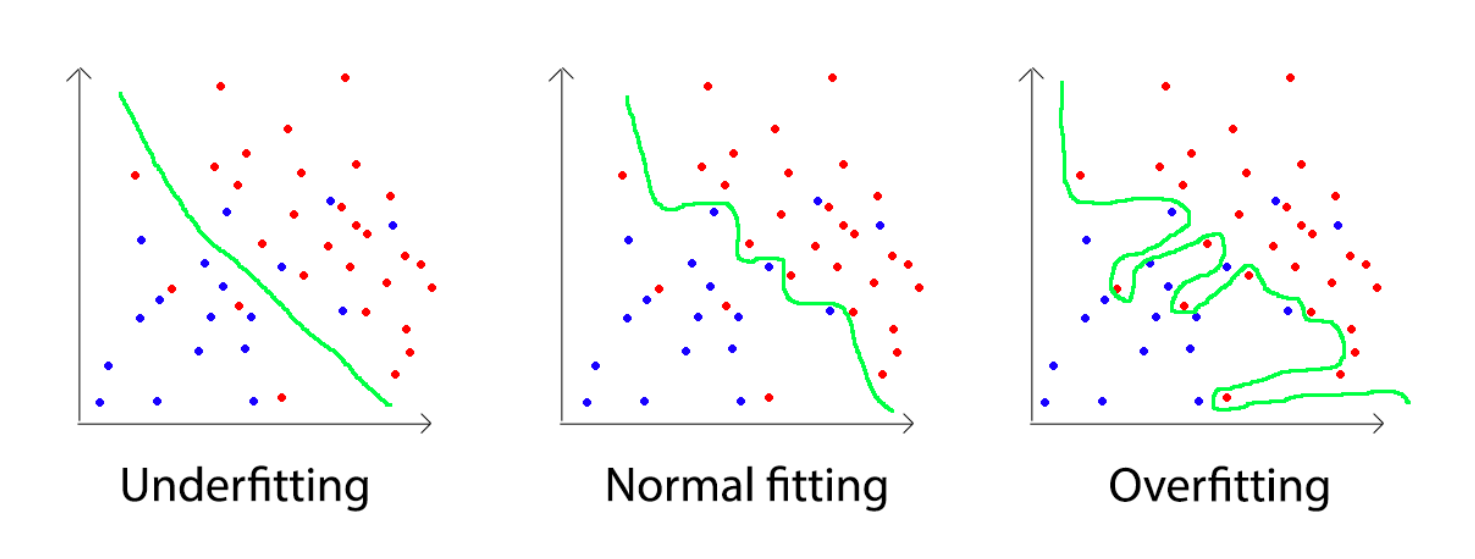• Underfitting, the model is too simple (for example, a linear model) and fails to grasp the complexity of the dataset
• Fitting appropriate, the model grasps the general "idea" of how the data is distracted, which does not try to change too much to represent each point
• Overfitting, the model modifies itself heavily to represent every point, but consequently "memorizes" the data of training, struggling, therefore, to generalize later.

Another important aspect to consider is the "price" of errors in prediction: classify as spam a regular email is very different from wrongly detecting cancer in an image, so the model performance, deriving from this tradeoff, is a key factor.

# Conclusions

Hopefully, now you should be more comfortable with high-level ideas of how ML systems are classified and you should have a clear idea of what the classification and regression tasks are.

Now you're ready to start your real Data Science Journey in the Purgatorio!